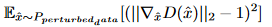# Out of memory with DRAGAN

Hello! I’m trying to reproduce this paper in code. It uses combination of losses for training GAN. One of the losses I need is DRAGAN loss. On every iteration of GAN lerning process I compute this loss, add it other losses and finally run backwards. Code for loss is:

``````            images = FloatTensor(get_data_minibatch())
p_images = images + 0.5 * torch.std(images) * torch.rand(images.size()).cuda()
p_images = torch.clamp(p_images, -0.99, 0.99)
D_p_images = discriminator(p_images)